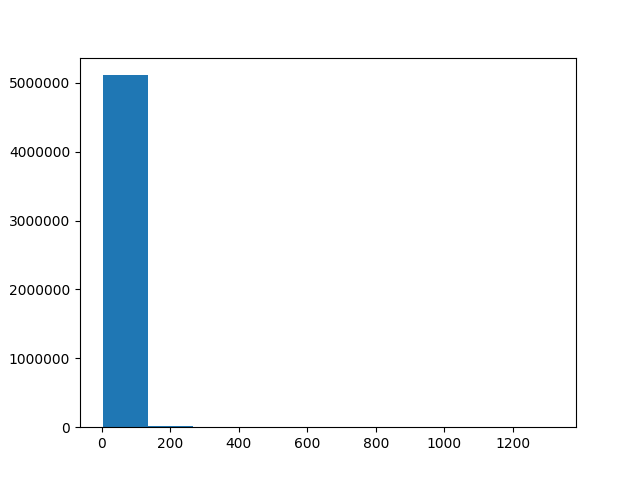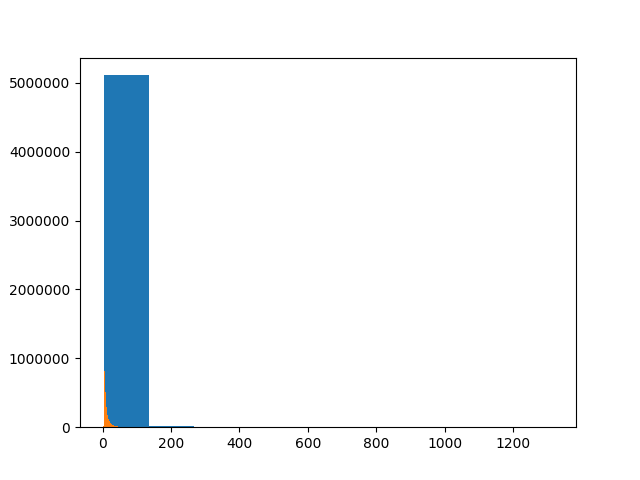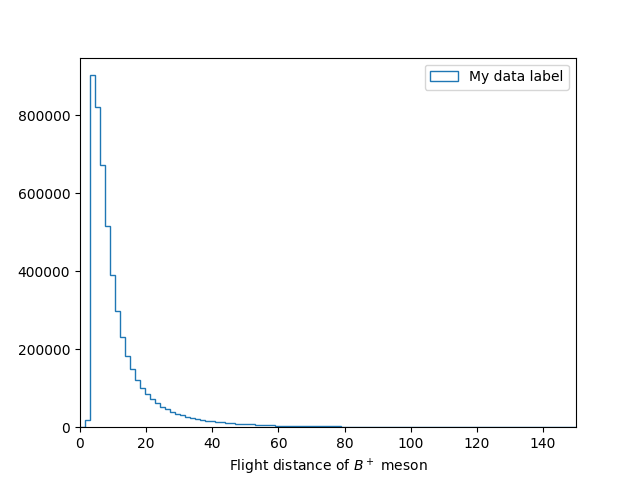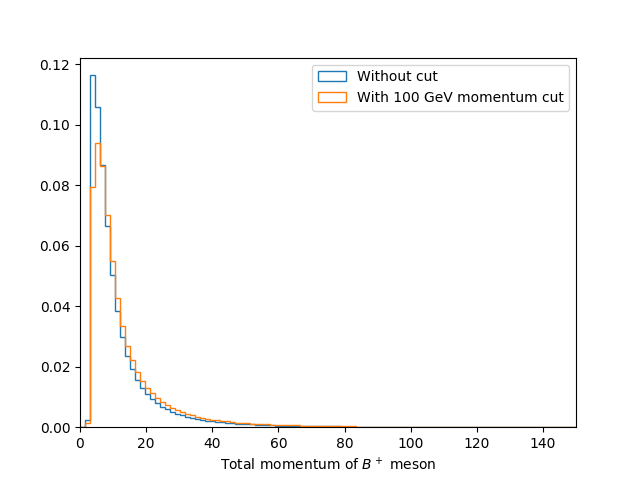In this section we are going to make our first plots using Python. For this we will use some public LHCb data for $$ B^+ \rightarrow H^+ H^+ H^- $$ from the CERN open data portal. This data is available on EOS:

$eos root://eospublic.cern.ch/ ls /eos/opendata/lhcb/AntimatterMatters2017/data B2HHH_MagnetDown.root B2HHH_MagnetUp.root PhaseSpaceSimulation.root  Installing python packages inside a virtual environment It is however usually preferable and safer to do everything inside a virtual environement. The latter is like a copy of your current environement. Thus you can modify your virtual environement (including installing/deleting/updating modules) without affecting your default environement. If at some point you realize you have broken everything, you can always exit the virtual environement and go back to the default lxplus one. To enter a suitable environment you can use: lb-conda default bash  You can then install stuff with pip. In this lesson we will be using root_pandas, which can be installed using: pip install --upgrade root_pandas matplotlib python -c 'import pandas; print(f"Got pandas from {pandas.__file__}")' python -c 'import root_pandas; print(f"Got root_pandas from {root_pandas.__file__}")' python -c 'import matplotlib; print(f"Got matplotlib from {matplotlib.__file__}")'  You can go back to the default environement using the deactivate command. Even if not recommended, you can also install modules directly, without using a virtual environment. While LCG provides a lot of useful packages, there are sometimes things missing. Fortunately, most packages can be easily installed into ~/.local/ using pip with the --user flag. In this lesson we will be using root_pandas, which can be installed using pip install --user root_pandas  Some packages, such as flake8, provide executables which are useful to have included on your PATH so they are available without specifying their absolute path. This can be done by running export PATH=$(python -m site --user-base)/bin:$PATH  see the bash lesson for more details about the PATH variable. We can even upgrade already installed packages using: pip install --user matplotlib --upgrade  As LCG uses PYTHONPATH to make packages available, any packages it provides have higher priority than the user installed packages. In order to make it so that a package installed with --user takes precedence, you must run export PYTHONPATH=$(python -m site --user-site):$PYTHONPATH  every time you run the source command above. This is only needed when installing user packages to upgrade LCG provided ones. All ROOT methods made available in Python using a set of automatically generated bindings, known as PyROOT. These are all available by using import ROOT. For example if we want to create a TFile using the aforementioned data we can launch ipython and run: In : import ROOT In : filename = 'root://eospublic.cern.ch//eos/opendata/lhcb/AntimatterMatters2017/data/B2HHH_MagnetDown.root' In : my_file = ROOT.TFile.Open(filename) In : my_file.ls()  TNetXNGFile** root://eospublic.cern.ch//eos/opendata/lhcb/AntimatterMatters2017/data/B2HHH_MagnetDown.root TNetXNGFile* root://eospublic.cern.ch//eos/opendata/lhcb/AntimatterMatters2017/data/B2HHH_MagnetDown.root KEY: TTree DecayTree;1 Tree continaing data for B- --> h-h+h- decays In : my_tree = my_file.Get('DecayTree') In : my_tree.Print() ****************************************************************************** *Tree :DecayTree : Tree continaing data for B- --> h-h+h- decays * *Entries : 5135823 : Total = 945201357 bytes File Size = 666480138 * * : : Tree compression factor = 1.42 * ******************************************************************************  While it is very useful to be able to access all the ROOT functionality in this way, they are often slow and tedious to use. Luckily people have built more specialised bindings to allow ROOT to be used in a more pythonic way. ## Pandas pandas is a library for doing data analysis on tabular data, using a data structure known as dataframes. These typically have columns with labels (like TLeafs in a TTree) with many rows. The root_pandas package we installed earlier can be used to create a pandas DataFrame from a root file: In : import root_pandas In : df = root_pandas.read_root(filename, key='DecayTree')  We can then use df.head(5) to see the first 5 rows of the DataFrame: In : df.head(5) Out: B_FlightDistance B_VertexChi2 H1_PX H1_PY H1_PZ \ 0 25.301004 1.497280 375.284205 831.308481 51820.233718 1 94.690700 1.383338 -4985.130785 5853.750057 326157.454706 2 8.284490 5.187101 -1265.456544 2330.050788 90762.658032 3 5.590769 7.129099 -720.797259 3413.790588 86793.058768 4 3.013242 10.988701 397.754571 1791.373059 40040.364159  While it is nice to be able to view the data, it is typically much more useful to be able to apply operations to it in bulk. In this example we have the momentum components for each of the child particles in the decay (H1, H2 and H3) but not the transverse momentum. We can however apply expressions to each row of the data like so: In : df.eval('sqrt(H2_PX**2 + H2_PY**2)') Out : 0 1306.642724 1 167.578904 2 1273.457019 3 1146.299204 ... 5135820 210.430531 5135821 762.344570 5135822 1454.471057 dtype: float64  This gives us the transverse momentum for each particle as an array, however, we can also create a new column with this information by assigning to the name we want to create and doing the action “inplace”: In : df.eval('H2_PT = sqrt(H2_PX**2 + H2_PY**2)', inplace=True)  Adding the total B+ meson momentum Create a new column in the dataframe called B_P for the total momentum of the B+ meson. Solution df.eval('B_P = sqrt(' '(H1_PX + H2_PX + H3_PX)**2 + ' '(H1_PY + H2_PY + H3_PY)**2 + ' '(H1_PZ + H2_PZ + H3_PZ)**2' ')', inplace=True)  ## Plotting histograms Now that we have the momentum of the $$ B+ $$ meson, it would be useful to plot its distribution in a histogram. We could use ROOT for this but the most popular Python library for plotting is known as matplotlib and this is what we will use here. The most common way matplotlib is used is with the pyplot interface imported as plt like so: In : import matplotlib In : matplotlib.use('Agg') # Force matplotlib to not use any Xwindows backend. In : from matplotlib import pyplot as plt In : plt.hist(df['B_FlightDistance']) In : plt.savefig('B_flight_distance.pdf')Interactive plotting There are various ways of viewing plots interactively such as: • plt.show() Opens a window with the current plot and pauses the Python interpreter until the window is closed • plt.ion() Allows plots to be viewed without pausing the Python interpreter • jupyter A web based interface for running various languages including python in “notebooks”. If you’ve used mathematica or matlab before it’s a similar interface with code, documentation and plots all shown together. In : import numpy as np In : bins = np.linspace(0, 150, 100) In : plt.hist(df['B_FlightDistance'], bins=bins) In : plt.savefig('B_flight_distance_v2.pdf')That’s not right! The new histogram has been plotted on top of our first one! This is normally useful as it allows us to layer plots on top of each other to compare them. Though in this case we don’t want to do that so we should first close the previous plot before making our histogram. We should also add axis labels and a legend like so: In : plt.close() # Close the previous plot In : plt.hist(df['B_FlightDistance'], bins=bins, histtype='step', label='My data label') In : plt.xlim(bins, bins[-1]) In : plt.xlabel('Flight distance of$B^+$meson') In : plt.legend(loc='best') In : plt.savefig('B_flight_distance_v3.pdf')## Applying cuts When analysing data it is often useful to “throw away” some data in order to in order change the contributions of a sample. Most commonly this is to remove some background(s) so we can more precisely study a process of interest. This is known as cutting. In pandas we can apply a cut to a DataFrame using the query method. For example to make a new DataFrame containing $$ B^+ $$ mesons with flight distances of more than 15 mm we can use: In : df_with_cut = df.query('B_FlightDistance > 15')  Comparing momentum distributions It is often useful to compare the effect of a cut on the distribution of another variable. Try to plot a histogram showing the B+ lifetime distribution with and without a total B+ momentum cut of 100000 MeV. Solution bins = np.linspace(0, 150, 100) df_with_cut = df.query('B_P > 100000') plt.figure() plt.hist(df['B_FlightDistance'], bins=bins, histtype='step', density=1, label='Without cut') plt.hist(df_with_cut['B_FlightDistance'], bins=bins, histtype='step', density=1, label='With 100 GeV momentum cut') plt.xlim(bins, bins[-1]) plt.xlabel('Total momentum of$B^+\$ meson')
plt.legend(loc='best')
plt.savefig('B_flight_distance_with_cut_compare.pdf')


Here we also use the normed=True option with plt.hist, this plots the normalised distribution. This is typically more useful when comparing distributions as it make it easier to see differences in the shape without the independently of the total number of events.Comparing momentum distributions

Calculate and plot the B+ meson mass assuming that all three of the child hadrons are kaons.